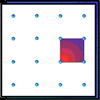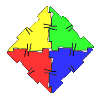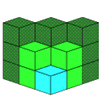#### You may also like### Geoboards

This practical challenge invites you to investigate the different squares you can make on a square geoboard or pegboard.### Polydron

This activity investigates how you might make squares and pentominoes from Polydron.### Multilink Cubes

If you had 36 cubes, what different cuboids could you make?

# Seven Flipped

##### Age 7 to 11Challenge Level

Joe from Bishop Ramsey School looked at the seven mat problem. He said:

In this problem it doesn't matter where you start on the diagram. My solution is written in a number of stages:
1) pick any 3 hexagons (they will turn blue)
2) pick 2 more hexagons and then pick one that you chose in the first go. You should now have 4 hexagons blue.
3) finally pick the three remaining red hexagons.
It would only have taken you 3 goes.

Many of you answered this part well, including Alistair of Cottenham Primary School.

Kahlia and Amy from Ardingly College Junior School then looked a bit further and tried with other numbers of mats:

If the number of tiles is a multiple of 3 it will divide equally into the number of tiles
eg: for 60 tiles, remove three each go: 60 divided by 3 = 20 turns

Amelia and Kathryn, also from Ardingly College Junior School, investigated many different numbers of mats in a very systematic way:

6= 2 moves
7= 3 moves
8= 4 moves
9= 3 moves
10= 4 moves
11= 5 moves
12= 4 moves
13= 5 moves
14= 6 moves
15= 5 moves
16= 6 moves
17= 7 moves
18= 6 moves
19= 7 moves
20= 8 moves

Kahlia and Amy identified a pattern:

If there is a number of tiles 1 more than a multiple of 3 you add 1 to the answer of the multiple below it eg: 18 tiles = 6 turns; 21 tiles = 7 turns
17 tiles = 7 turns; 20 tiles = 8 turns

Jeff and Raphael from Zion Heights Junior High School relate this back to the strategy for flipping the mats:

For numbers with one remainder after dividing by three, you follow the strategy for 7 mats stated above.
For numbers with 2 remainders, you flip over two mats instead of one on the second move, thus taking one more move.

So, thinking about this like Kahlia and Amy did, we could say that if the number of tiles is 2 more than a multiple of 3, you add 2 to the answer of the multiple below it.

Well done to everyone who tackled this problem - it wasn't easy at all.